Estimation of the Residual Stress in Clay Sampled from the Field | OMICS International
Journal of Civil & Environmental Engineering

# Estimation of the Residual Stress in Clay Sampled from the Field

 Owayo AA* and Ou CY Department of Civil and Construction Engineering, National Taiwan University of Science and Technology, ROC Taipei-Taiwan Corresponding Author : Owayo AA Department of civil and construction engineering National Taiwan University of science and Technology ROC Taipei-Taiwan Tel: +886 2 2733 31 E-mail: [email protected] Received May 12, 2014; Accepted June 17, 2014; Published June 23, 2014 Citation: Owayo AA, Ou CY (2014) Estimation of the Residual Stress in Clay Sampled from the Field. J Civil Environ Eng 4: 149. doi: 10.4172/2165-784X.1000149 Copyright: © 2014 Owayo AA, et al. This is an open-access article distributed under the terms of the Creative Commons Attribution License, which permits unrestricted use, distribution, and reproduction in any medium, provided the original author and source are credited.

Visit for more related articles at Journal of Civil & Environmental Engineering

#### Abstract

The objective of this study is to establish a reliable way to determine the residual stress in clay sampled from the field. During saturation in triaxial test, using residual stress as back pressure as opposed to the traditional saturation methods leads to better sample quality. In this research the residual stress within the sample is measured and compared against the one that can be predicted with the help of the equation proposed by Skempton based on the account of change in total stress during sampling. The results do not exhibit any predictable trend, in fact sometimes the prediction is quite good while the rest of the time not good. Thus in the absence of a perfect sample, the use of Skempton’s equation is more reliable.

 Keywords Residual stress; Triaxial test; Skempton’s equation; Clay; Perfect sample; Sampling; Saturation Introduction During triaxial testing we require a sample with as minimal disturbance as possible. The in-situ conditions needs to be preserved as best as possible. Residual effective stress also called the initial mean effective stress, stored effective stress or effective stress after sampling is the effective stress remaining in the soil sample after sampling, handling and storage. This is a concept rooted in perfect sampling concept where there would be no disturbance except that from stress relief. The difference between sampling effective stress and the residual effective stress is caused by disturbance rather than stress relief. This implies that the residual stress can be a qualitative measure of sample disturbance . The sample swelling thus disturbance during saturation, can be minimized by applying an effective stress equal to the measured residual stress before adding water to the specimen . This implies that the sample quality may be improved further if the correct or ‘true’ residual stress is used. This informed by the fact that due to poor storage, and handling the sample may undergo pore pressure dissipation and hence the measured residual stress is not the ‘true’ value. The measured residual stress is applied as back pressure during saturation. In the conventional saturation process, usually very small back pressure is used; this could lead to sample swelling thus disturbance. Skempton proposed an equation that may be used to estimate residual effective stress within a sample based on the account of total stress changes, equation 1. Equation 2 may be used to compute the pore water pressure change on the account of total stress change. This research seeks to measure the remaining residual stress in the in situ sample and compares it against the prediction from equation 2. Certain prediction matches the experimental values so well as elucidated in Figure 3 while others do not. Practically speaking, it is difficult to eliminate the sources of disturbance. Inevitable disturbance may be encountered during sample extrusion, trimming etc. Non homogeneity of the soil i.e. presence of foreign material like silt or sand may lead to pore water pressure dissipation thus the measured residual stress is not the ‘true’ residual stress or the expected residual stress immediately after sampling. Since the residual stresses experimentally determined from the laboratory depends on uniformity of the sample and human factor such as sample handling, storage, trimming etc. The effects of disturbance due to these factors are difficult to quantify. Method Soil description Tube samples of Taipei silty clay sampled from within the environs of Taipei 101 were used. Taipei Silty clay has its water table at 2 meters below the surface. The basic soil properties are as summarized below in Table 1. • Unified soil classification system (USCS) • Natural water content, Wn • Liquid limit, LL • Plastic, limit, PL • Plastic index, PI • Shrinkage Limit SL • Bulk density γt • Coefficient of swelling, Cs • Coefficient of recompression, Cr • σ1 is total vertical stress • σ3 is horizontal total stress • γsat is saturated unit weight of the soil • γw is the unit weight of water •z is the sample depth. Idealized concept of stress relief during sampling and the consequent pore water pressure changes Δu = ΒΔσ3 + A(Δσ1− Δσ3) (1)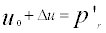(2) A and B are Skemptons pore pressure parameters The equation 1 as proposed by Skempton in 1954 can be used to estimate the changes due to stress relief. k0 for saturated soil is taken as 0.5, B=1 and A=2/3 for axial extension synonymous to unloading during sampling . Before sampling as shown in Figure 1, the soil sample experiences a typical triaxial compression test condition , an overburden total stress, σ1 and a horizontal total stress, σ3 the soil also experiences a hydrostatic water pressure, u0. After sampling, the soil sample is under no confinement but is still standing this implicitly means that there is a negative pore water pressure, -ur in the sample preventing it from collapsing. This is theoretically equals the residual stress in the sample. The resultant change in pore water pressure Δu, may be estimated by u0-ur. Δu may also be estimated from equation 1. In the laboratory we are able to measure -ur, ideally this value is the residual stress immediately after sampling: but due to disturbance during sampling handling, pore water dissipation and storage this value may be less than the expected value. Theoretically when the estimation from equation 2 equals the measured residual stress in the laboratory, this implies that the sample has not experienced substantial disturbance hence is close to a perfect sample. The residual stress is the intercept of Y axis (negative value) when the cell pressure equal zero. Residual stress measurement The effective stress, p’r , was evaluated prior to saturation through the response of excess pore-water pressure as isotropic stress increaments applied with drainage lines closed. The total confining stress, σc was increased in 50 kPa from 100 kPa to 300 kPa and reduced in 100 kPa from 300 kPa to 100 kpa. In each load increament step the pore water, u, was allowed to equillibrate within 30-60 min. The same procedure was followed in reducing σc. Expected response of u to σc is as shown in (Figure 2). Linear regression applied to this data, the matric suction within the sample can be taken as th e value of u at σc=0. Following the general concept of effective stress,the residual effective stress, p’r may be defined: p’r = -u r (3) Results VCL, is the virgin consolidation line Quantifying the levels of disturbance Figure 3 is established from the fact that, we know the in-situ preconsolidation stress and the original void ratio can be estimated from the in situ water content for each sample. This point on the VCL can thus be established. The gradient of VCL is the value of Cc. Recompression of the sample follows the value of Cr (Figure 4). Measured residual effective stress against the estimation from equation Summary of measured residual stress against the estimation from Skempton’s formula is shown in Table 2. Discussion Sampling relieves the sample of its overburden pressure effectively reducing the in-situ pre-consolidation stress to zero as shown in Figure 3. The sample is not allowed to swell since it is confined by the sample tube. If there is no disturbance, we expect no volumetric changes thus constant void ratio, equal to the field void ratio. A negative pore water pressure equal to the residual stress is developed in the sample to prevent it from collapsing and help it maintain its void ratio. However if any disturbance is caused to the sample this may result in volumetric changes thus a change in the void ratio. Traditional saturation method uses very small back pressure, normally close to zero kPa as shown in Figure 3. When a very small back pressure is used during saturation to recompress the sample back to the in-situ condition before triaxial test is done on the sample. A path similar to the one shown in dotted green line is followed. On the other hand, if measured residual stress (usually higher than zero) is used during saturation to recompress the sample back to the in-situ state. A path similar to the solid pink line is followed. Recompressing the soil sample back to the original in situ state leads to volumetric changes in the sample thus the changes in void ratio as in Figure 3. The subsequent changes in void ratio when traditional saturation is employed Δe2, is much higher than the changes in void ratio Δe1 when measured residual stress is used as back pressure. Figure 3 also clearly indicates that when the measured residual stress is used as back pressure: when the consolidation stress equal to or higher than the in-situ pre-consolidation stress, if residual stress is used for saturation then the sample is much closer to the virgin consolidation line compared to when traditional saturation technique is applied, leading to better sample quality. For all the samples used in this study we established (Figure 3) for each sample, from which we derived (Table 3). When the consolidation stress is higher or equal to the in-situ pre-consolidation stress the soil sample is at the virgin consolidation line (VCL), where it is normally consolidated. The prediction from equation 2 matches the measured residual stress in samples 1 and 4 very well. While for samples 2 and 3 there is a substantial difference between the prediction of equation 2 and the measured value i.e. the measured value is much smaller. This difference may be attributed to the possible accumulated effects of disturbance in the course of soil handling from the sampling stage, storage and performing the test. Presence of foreign material in the clay such as silt or sand may also make it easier for the dissipation of pore water pressure during sample extrusion and trimming so that the measured residual stress is way below the expected ‘true value’ . Thus the measured residual stress may not be the actual, correct or the ‘true’ residual. When the measured residual stress is used the volumetric changes are far much less as compared to when traditional saturation process is employed . The classification of sample quality ranges from ‘Fair to Poor’ to Very good when residual stress is used as back pressure (Table 4). When conventional saturation is used as back pressure during saturation the classification is from ‘Very Poor’ to ‘Fair to Poor’. It is expected thus, that if the ‘true’ residual stress estimated from equation 2 is used all the samples would fall within the ‘Very Good’ quality classification. It is clear thus that when the residual stress is used as back pressure during the saturation stage the sample quality is much more improved (Figure 5). CIU_AC-Isotropic consolidated undrained axial compression test These results demonstrate that the use of Skemptons equation to predict residual stress agrees very well with the experimental data when the sample in question has the insitu conditions preserved as best as possible. While when the prediction does not agree with the experimental value; this is possibly due to disturbance in the course of soil handling,storage trimming, extrusion etc. Since all the samples used here in were stored within the same environment, and all the experiments carried out by the same individual. It is expected that the accumulated effects of disturbance that may be attributed to the environment, human judgement,method of sample preparation etc. are fairly controlled and are the same for all the samples. Employing the same procedure of sample preparation and experimental execution some samples turn out to give estimates close to equation 2 while others do not. We therefore deduce that this may be due to disturbance, and it’s effects to the remaining residual stress in the sample. These effect appear to be random and are therefore difficult to quantify. This further implies that the samples whose prediction from equation 2 and measured values from the experiment are close, are very good quality samples with minimal disturbance. The samples whose prediction and measurement do not agree implies disturbance to the samples and thus are samples of lesser quality. Conclusion When measured residual stress is used as back pressure during saturation in the triaxial test the sample quality is quite improved. If the sample is disturbed, pore water pressure dissipates in the course of sample handling thus, the measured residual stress is not the actual or the expected ‘true’ residual value. While when the true residual stress is used the sample quality is expected to be much more improved. The use of equation 2 to estimate the residual stress would be more reliable in cases where a sample is relatively disturbed. References Cho W, Terence P, Holman, Jung YH, Finno RJ (2006). Effects of Swelling During Saturation in Triaxial Test in Clays. Geotechnical testing Journal. Holtz RD, Kovacs WD (1991) An introduction to Geotechnical Engineering: Prentice-Hall. Standard Test Method for Consolidated Undrained Triaxial Compression Test for Cohesive Soils. ASTM D4767 – 11. Das BM (1978) Advanced Soil Mechanics. Taylor and Francis. Teng FC, Ou CY (2011) Application of a Suction Control System in the Method of Specimen Saturation in Triaxial Tests. Geotechnical testing Journal.

#### Tables and Figures at a glance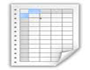Table 1 Table 2 Table 3 Table 4

#### Figures at a glance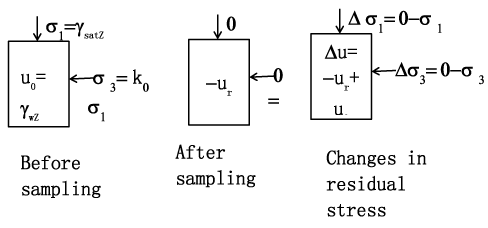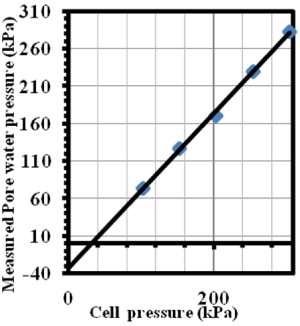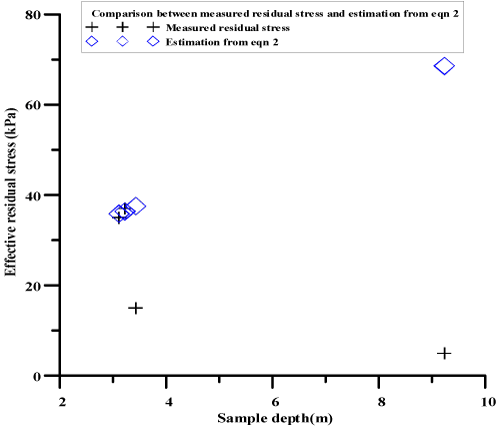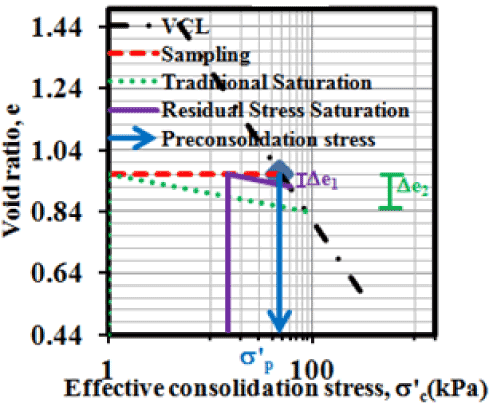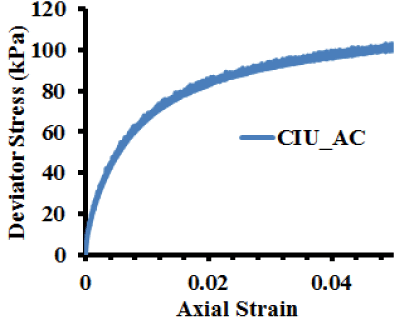Figure 1 Figure 2 Figure 3 Figure 4 Figure 5
Select your language of interest to view the total content in your interested language

### Article Usage

• Total views: 12636
• [From(publication date):
October-2014 - Jan 24, 2020]
• Breakdown by view type
• HTML page views : 8786Can't read the image? click here to refresh